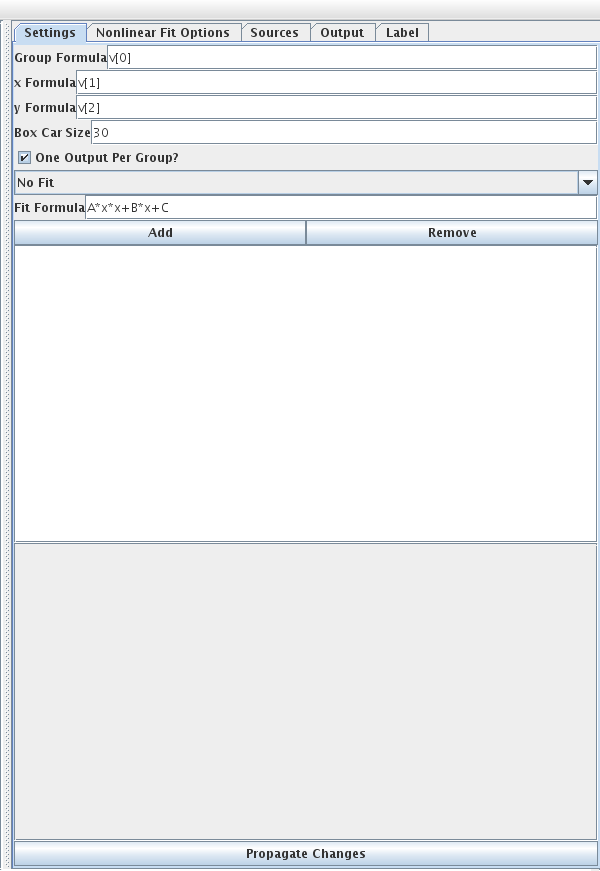# Box Car Filter

This filter allows a variety of processing on data with a general theme that data elements are taken in a group that slides across. The simplest usage of this is to do a box car averaging. In addition to taking a simple average, you can also do fits to the data in the group and take information from the fit values. The image below shows the properties for this filter.The first setting is a group formula that is used when the data is broken into groups. This is useful when the data is not a single long sequence, but a set of different sequences. After that you get to enter formulas for x and y. You should be thinking of this as y(x). The box car size tells the filter how many consecutive elements the box car should cover. If you select the "One Output Per Group?" option then only one element will be added for each group instead of one element for each box car.

By default, the filter does not do a fit. In this setting each box car will give you access to the values aveX, aveY, minX, minY, maxX, maxY, stdX, and stdY. If you set it to do a fit, the fit formula should be entered in. The default formula is a quadratic with constants A, B, and C. When you do a fit, the fit constants can also be used in the outputs.

The last thing you have to set is the outputs. There are three types of outputs currently in the filter and more can be added if you have one that makes sense. The three types are basic, average, and sign switch. The basic output type is most useful when you are doing an output for each box car. For each of these you can specify a name, formula, condition, and whether this output should be a parameter. The formula can use any of the variables you get without a fit as well as those you get with a fit. If you use a variable or a parameter it will be evaluated for the first element in the box car. The condition will allow you to determine when something gets output. For example, you might want aveX for the formula, but only if maxX>1e5.

The average style output has the same settings as basic, but it will accumulate an average across all the box cars in a group for every box car that passes the conditional. If you use this, make sure that you have selected one output per group.

The sign switch style has more options. It should also be used with one output per group. This style runs through a group and keeps track of a specified formula, the sign formula, to see when ti switches signs. Anytime the formula is above a specified magnitude and it changes sign, a new value is added to the element, up to a specified maximum number. The value that gets added is based on the combination of two formulas. The last option you select is how those two formulas are combined.